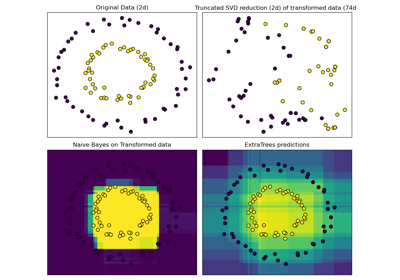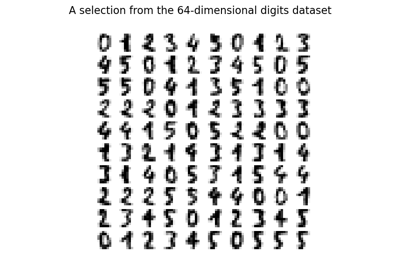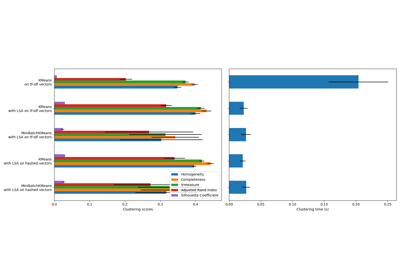# sklearn.decomposition.TruncatedSVD¶

class sklearn.decomposition.TruncatedSVD(n_components=2, *, algorithm='randomized', n_iter=5, n_oversamples=10, power_iteration_normalizer='auto', random_state=None, tol=0.0)[source]

Dimensionality reduction using truncated SVD (aka LSA).

This transformer performs linear dimensionality reduction by means of truncated singular value decomposition (SVD). Contrary to PCA, this estimator does not center the data before computing the singular value decomposition. This means it can work with sparse matrices efficiently.

In particular, truncated SVD works on term count/tf-idf matrices as returned by the vectorizers in sklearn.feature_extraction.text. In that context, it is known as latent semantic analysis (LSA).

This estimator supports two algorithms: a fast randomized SVD solver, and a “naive” algorithm that uses ARPACK as an eigensolver on X * X.T or X.T * X, whichever is more efficient.

Read more in the User Guide.

Parameters:
n_componentsint, default=2

Desired dimensionality of output data. If algorithm=’arpack’, must be strictly less than the number of features. If algorithm=’randomized’, must be less than or equal to the number of features. The default value is useful for visualisation. For LSA, a value of 100 is recommended.

algorithm{‘arpack’, ‘randomized’}, default=’randomized’

SVD solver to use. Either “arpack” for the ARPACK wrapper in SciPy (scipy.sparse.linalg.svds), or “randomized” for the randomized algorithm due to Halko (2009).

n_iterint, default=5

Number of iterations for randomized SVD solver. Not used by ARPACK. The default is larger than the default in randomized_svd to handle sparse matrices that may have large slowly decaying spectrum.

n_oversamplesint, default=10

Number of oversamples for randomized SVD solver. Not used by ARPACK. See randomized_svd for a complete description.

New in version 1.1.

power_iteration_normalizer{‘auto’, ‘QR’, ‘LU’, ‘none’}, default=’auto’

Power iteration normalizer for randomized SVD solver. Not used by ARPACK. See randomized_svd for more details.

New in version 1.1.

random_stateint, RandomState instance or None, default=None

Used during randomized svd. Pass an int for reproducible results across multiple function calls. See Glossary.

tolfloat, default=0.0

Tolerance for ARPACK. 0 means machine precision. Ignored by randomized SVD solver.

Attributes:
components_ndarray of shape (n_components, n_features)

The right singular vectors of the input data.

explained_variance_ndarray of shape (n_components,)

The variance of the training samples transformed by a projection to each component.

explained_variance_ratio_ndarray of shape (n_components,)

Percentage of variance explained by each of the selected components.

singular_values_ndarray of shape (n_components,)

The singular values corresponding to each of the selected components. The singular values are equal to the 2-norms of the n_components variables in the lower-dimensional space.

n_features_in_int

Number of features seen during fit.

New in version 0.24.

feature_names_in_ndarray of shape (n_features_in_,)

Names of features seen during fit. Defined only when X has feature names that are all strings.

New in version 1.0.

DictionaryLearning

Find a dictionary that sparsely encodes data.

FactorAnalysis

A simple linear generative model with Gaussian latent variables.

IncrementalPCA

Incremental principal components analysis.

KernelPCA

Kernel Principal component analysis.

NMF

Non-Negative Matrix Factorization.

PCA

Principal component analysis.

Notes

SVD suffers from a problem called “sign indeterminacy”, which means the sign of the components_ and the output from transform depend on the algorithm and random state. To work around this, fit instances of this class to data once, then keep the instance around to do transformations.

References

Halko, et al. (2009). “Finding structure with randomness: Stochastic algorithms for constructing approximate matrix decompositions”

Examples

>>> from sklearn.decomposition import TruncatedSVD
>>> from scipy.sparse import csr_matrix
>>> import numpy as np
>>> np.random.seed(0)
>>> X_dense = np.random.rand(100, 100)
>>> X_dense[:, 2 * np.arange(50)] = 0
>>> X = csr_matrix(X_dense)
>>> svd = TruncatedSVD(n_components=5, n_iter=7, random_state=42)
>>> svd.fit(X)
TruncatedSVD(n_components=5, n_iter=7, random_state=42)
>>> print(svd.explained_variance_ratio_)
[0.0157... 0.0512... 0.0499... 0.0479... 0.0453...]
>>> print(svd.explained_variance_ratio_.sum())
0.2102...
>>> print(svd.singular_values_)
[35.2410...  4.5981...   4.5420...  4.4486...  4.3288...]


Methods

 fit(X[, y]) Fit model on training data X. fit_transform(X[, y]) Fit model to X and perform dimensionality reduction on X. get_feature_names_out([input_features]) Get output feature names for transformation. get_params([deep]) Get parameters for this estimator. Transform X back to its original space. set_output(*[, transform]) Set output container. set_params(**params) Set the parameters of this estimator. Perform dimensionality reduction on X.
fit(X, y=None)[source]

Fit model on training data X.

Parameters:
X{array-like, sparse matrix} of shape (n_samples, n_features)

Training data.

yIgnored

Not used, present here for API consistency by convention.

Returns:
selfobject

Returns the transformer object.

fit_transform(X, y=None)[source]

Fit model to X and perform dimensionality reduction on X.

Parameters:
X{array-like, sparse matrix} of shape (n_samples, n_features)

Training data.

yIgnored

Not used, present here for API consistency by convention.

Returns:
X_newndarray of shape (n_samples, n_components)

Reduced version of X. This will always be a dense array.

get_feature_names_out(input_features=None)[source]

Get output feature names for transformation.

The feature names out will prefixed by the lowercased class name. For example, if the transformer outputs 3 features, then the feature names out are: ["class_name0", "class_name1", "class_name2"].

Parameters:
input_featuresarray-like of str or None, default=None

Only used to validate feature names with the names seen in fit.

Returns:
feature_names_outndarray of str objects

Transformed feature names.

get_params(deep=True)[source]

Get parameters for this estimator.

Parameters:
deepbool, default=True

If True, will return the parameters for this estimator and contained subobjects that are estimators.

Returns:
paramsdict

Parameter names mapped to their values.

inverse_transform(X)[source]

Transform X back to its original space.

Returns an array X_original whose transform would be X.

Parameters:
Xarray-like of shape (n_samples, n_components)

New data.

Returns:
X_originalndarray of shape (n_samples, n_features)

Note that this is always a dense array.

set_output(*, transform=None)[source]

Set output container.

See Introducing the set_output API for an example on how to use the API.

Parameters:
transform{“default”, “pandas”}, default=None

Configure output of transform and fit_transform.

• "default": Default output format of a transformer

• "pandas": DataFrame output

• None: Transform configuration is unchanged

Returns:
selfestimator instance

Estimator instance.

set_params(**params)[source]

Set the parameters of this estimator.

The method works on simple estimators as well as on nested objects (such as Pipeline). The latter have parameters of the form <component>__<parameter> so that it’s possible to update each component of a nested object.

Parameters:
**paramsdict

Estimator parameters.

Returns:
selfestimator instance

Estimator instance.

transform(X)[source]

Perform dimensionality reduction on X.

Parameters:
X{array-like, sparse matrix} of shape (n_samples, n_features)

New data.

Returns:
X_newndarray of shape (n_samples, n_components)

Reduced version of X. This will always be a dense array.

## Examples using sklearn.decomposition.TruncatedSVD¶Hashing feature transformation using Totally Random Trees

Hashing feature transformation using Totally Random TreesManifold learning on handwritten digits: Locally Linear Embedding, Isomap…

Manifold learning on handwritten digits: Locally Linear Embedding, Isomap...Column Transformer with Heterogeneous Data Sources

Column Transformer with Heterogeneous Data SourcesClustering text documents using k-means

Clustering text documents using k-means